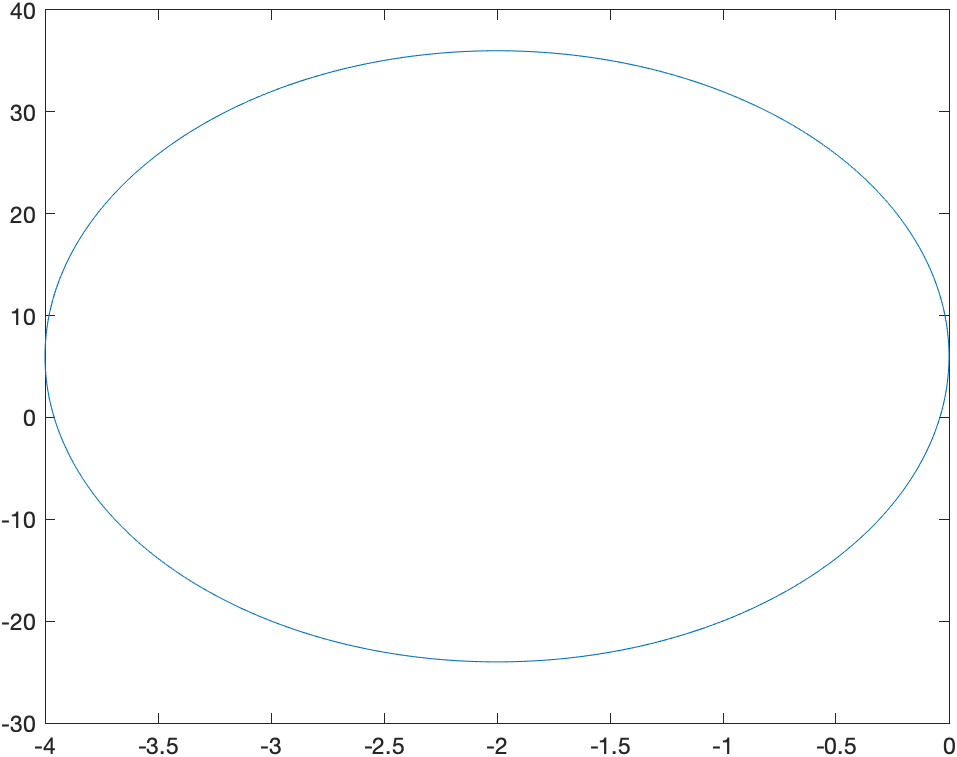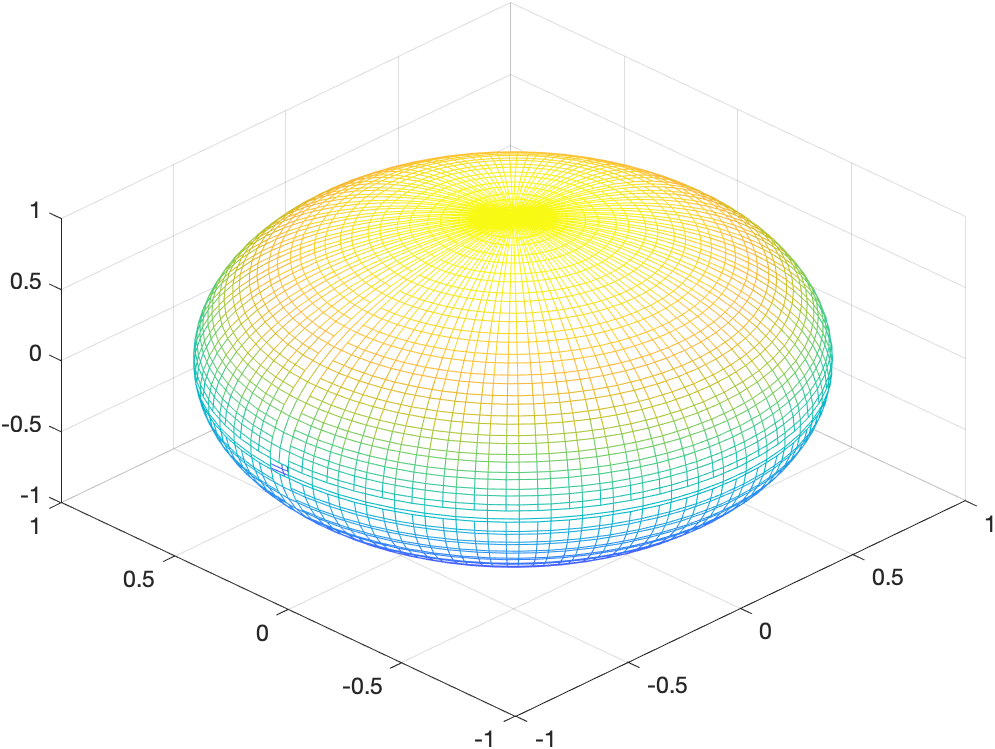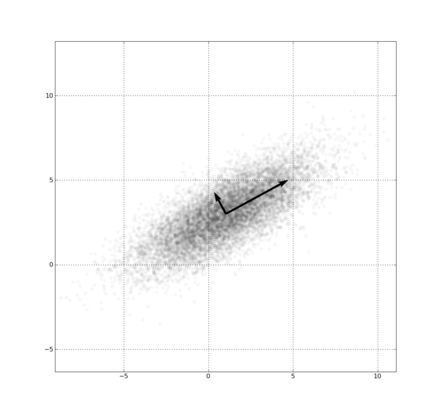# 数据降维：主成分分析法

01/19 00:34

## 前言## 思想## 在数学上

$c^*=(L^2)^2=argmin_c||x-g(c)||_2^2 \\ =(x-g(c))^T(x-g(c)) \\ =x^Tx-2x^Tg(c)+g(c)^Tg(c) \\ =argmin_c-2x^TDc+c^TI_lc \\ （其中c=f(x)，g(c)=Dc） \\ \therefore\nabla_c(-2x^TDc+c^Tc)=0 \\ c=f(x)=D^Tx$

$r(x)=g(f(x))=DD^Tx \\ D^*=argmin_D\sqrt{\sum_{i,j}(x_j^{(i)}-r(x^{(i)})_j)^2} \\ 其中D^TD=I_l$

## 总结

• 本文首发自RAIS

0
0 收藏

### 作者的其它热门文章0 评论
0 收藏
0